## Tamilnadu Samacheer Kalvi 6th Maths Solutions Term 3 Chapter 4 Geometry 4.1

Question 1.
Fill in the blanks
i) The reflected image of the letter ‘q’ is ____.
Hint: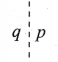Solution:
p

ii) A rhombus has ___ lines of symmetry.
Hint: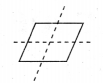Solution:
two

iii) The order of rotational symmetry of the letter ‘Z’ is ___
Solution:
two

iv) A figure is said to have rotational symmetry, if the order of rotation is atleast ___
Solution:
two

v) ___ symmetry occurs when an object slides to new position.
Solution:
TranslationQuestion 2.
Say True or False
(i) A rectangle has four lines of symmetry.
(ii) A shape has reflection symmetry if it has a line of symmetry.
(iii) The reflection of the name RANI is INAЯ.
(iv) Order of rotation of a circle is infinite.
(v) The number 191 has rotational symmetry.
Solution:
(i) False
(ii) True
(iii) False
(iv) True
(v) FalseQuestion 3.
Match the following shapes with their number of lines of symmetry.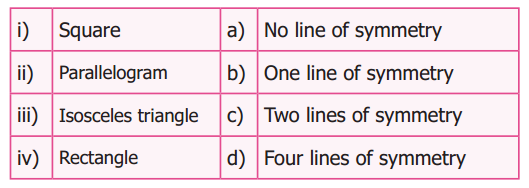Solution: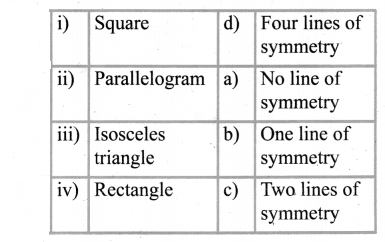Question 4.
Draw the lines of symmetry of the following.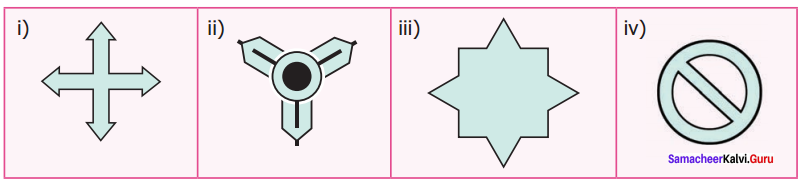Solution: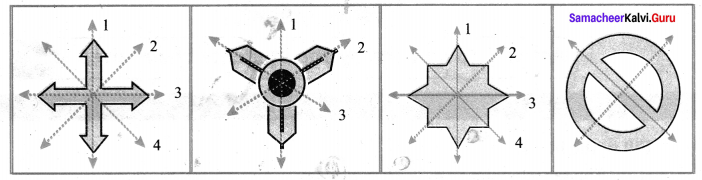Question 5.
Using the given horizontal line / vertical line as a line of symmetry, complete each alphabet to discover the hidden word.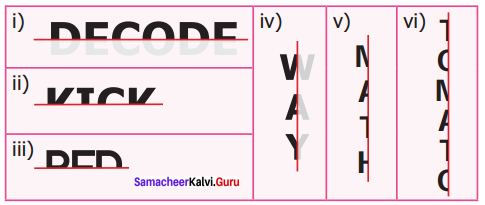Solution: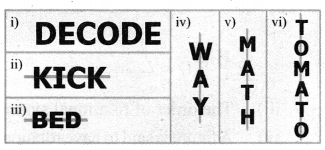Question 6.
Draw a line of symmetry of the given figures such that one hole coincide with the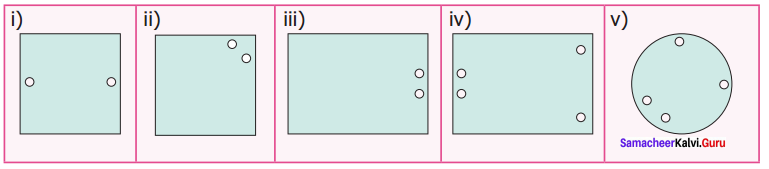Solution:
The lines of symmetry to coincide one hole with the other are given below.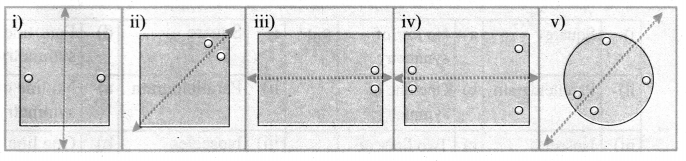Question 7.
Complete the other half of the following figures such that the dotted line is the line of Symmetry.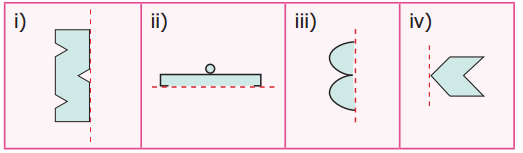Solution:
Completing the figures about the line of symmetry we get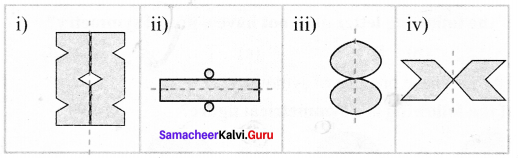Question 8.
Find the order of rotation for each of the following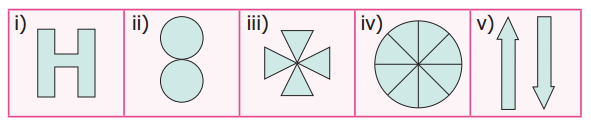Solution: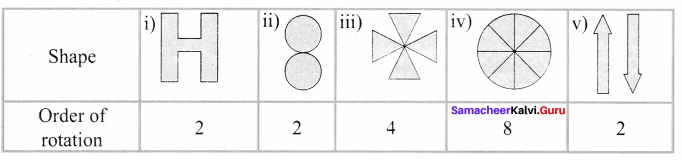Question 9.
A standard die has six faces which are shown below. Find the order of rotational symmetry of each face of a die?Solution:
The order of rotational symmetry of all faces of die are given below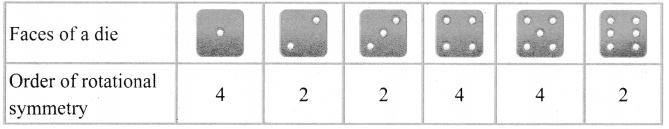Question 10.
What pattern is translated in the given border kolams?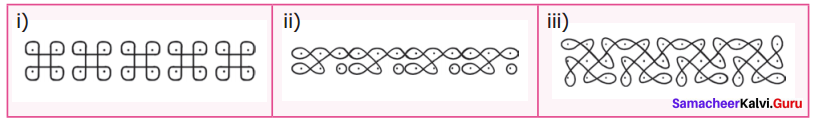Solution:
The pattern that translated in each diagrams are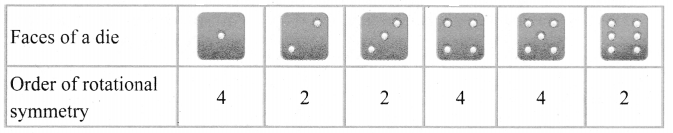Objective Type Questions

Question 11.
Which of the following letter does not have a line of symmetry?
(a) A
(b) P
(c) T
(d) U
Solution:
(b) PQuestion 12.
Which of the following is a symmetrical figure?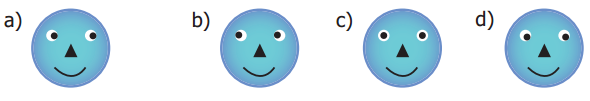Hint:
refer eyes
Solution: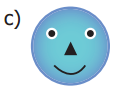Question 13.
Which word has a vertical line of symmetry?
(b) NUN
(c) MAM
(d) EVE
Solution:
(c) MAMQuestion 14.
The order of rotational symmetry of 818 is
(a) 1
(b) 2
(c) 3
(d) 4
Solution:
(b) 2

Question 15.
The order of rotational symmetry of * is ……….
(a) 5
(b) 6
(c) 7
(d) 8
Solution:
(a) 5